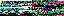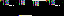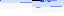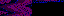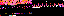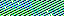EVR.Animation.Scroller = function(graphics, step, rate)
{
EVR.Animation.call(this, rate);
this.graphics = graphics;
this.step = step;
}
EVR.Animation.Scroller.prototype = new EVR.Animation;
EVR.Animation.Scroller.prototype.sequence = function()
{
var graphics = this.graphics;
var range = graphics.container.get_dimensions();
var current, width, x, difference, step = this.step * range;
for (var ii = 0, len = graphics.length; ii < len; ii++)
{
current = graphics[ii];
width = current.get_dimensions();
x = current.get_coordinates() - step;
if (x < -width)
{
difference = -x - width;
x = range - difference;
}
current.set_coordinates([x]);
}
}
EVR.Animation.Scroller.prototype.toString = function()
{
return "[object EVR.Animation.Scroller]";
}
EVR.Animation.Introduction.Black_Hole = function(introduction, rate)
{
var container = introduction.container;
var width = introduction.beam_width_ratio;
EVR.Beam.call(this, container, "black", width, null, null, ALIGN_TOP);
EVR.Animation.call(this, introduction.rate);
this.introduction = introduction;
this.entrance = introduction.entrance;
this.set_opacity(0);
this.place(0, introduction.offset);
}
EVR.Animation.Introduction.Black_Hole.prototype = new EVR.Beam;
EVR.inherit(EVR.Animation.Introduction.Black_Hole, EVR.Animation);
EVR.Animation.Introduction.Black_Hole.prototype.sequence = function(step)
{
var new_opacity = this.get_opacity() + step;
this.set_opacity(new_opacity);
if (new_opacity >= 1 || new_opacity <= 0)
{
this.stop();
if (new_opacity >= 1)
{
this.set_opacity(1);
this.next.play();
}
else
{
this.set_opacity(0);
var current = this;
window.setTimeout(
function()
{
current.introduction.play();
}, INTRODUCTION_RESET_DELAY);
}
}
}
EVR.Animation.Introduction.Black_Hole.prototype.remove = function()
{
this.stop();
if (this.attached)
{
EVR.Beam.prototype.remove.call(this);
}
}
EVR.Animation.Introduction.Black_Hole.prototype.toString = function()
{
return "[object EVR.Animation.Introduction.Black_Hole]";
}
EVR.Animation.Introduction.Entrance = function(
emoticon, jump, option_count, top_edge, bottom_edge, rate)
{
EVR.Animation.call(this, rate);
this.emoticon = emoticon;
this.jump = jump;
this.option_count = option_count;
this.top_edge = top_edge;
this.bottom_edge = bottom_edge;
this.distance = 0;
}
EVR.Animation.Introduction.Entrance.prototype = new EVR.Animation;
EVR.Animation.Introduction.Entrance.prototype.sequence = function()
{
var step = this.jump * INTRODUCTION_EMOTICON_WALK_SPEED;
this.distance += step;
this.emoticon.move(step, 0);
if (this.distance >= this.jump)
{
this.stop();
}
}
EVR.Animation.Introduction.Entrance.prototype.travel = function(boundary)
{
boundary -= this.emoticon.get_dimensions(true);
var step = this.jump * INTRODUCTION_EMOTICON_RUN_SPEED;
this.emoticon.move(step, 0);
var border = this.get_border();
if (border >= boundary)
{
this.emoticon.move(boundary - border);
this.stop();
}
}
EVR.Animation.Introduction.Entrance.prototype.get_border = function()
{
return this.emoticon.get_coordinates(true) +
this.emoticon.get_dimensions(true);
}
EVR.Animation.Introduction.Entrance.prototype.raise = function(black_hole)
{
var step = this.jump * INTRODUCTION_EMOTICON_FLOAT_SPEED;
this.emoticon.move(null, -step);
var y = this.emoticon.get_coordinates(true);
if (y <= this.top_edge)
{
this.stop();
this.emoticon.move(null, this.top_edge - y);
this.end(black_hole);
}
}
EVR.Animation.Introduction.Entrance.prototype.end = function(black_hole)
{
this.stop();
var y_offset = this.jump * this.option_count;
this.emoticon.range = black_hole;
this.emoticon.place(null, -y_offset);
this.offset();
}
EVR.Animation.Introduction.Entrance.prototype.offset = function()
{
this.emoticon.draw();
if (this.emoticon.range != this.emoticon.container)
{
var x_offset = this.emoticon.get_dimensions(true) * 2;
this.emoticon.place(-x_offset);
}
}
EVR.Animation.Introduction.Entrance.prototype.toString = function()
{
return "[object EVR.Animation.Introduction.Entrance]";
}
EVR.include("Beam.js");
EVR.include("animation/introduction/Black_Hole.js");
EVR.include("animation/introduction/transmission/Transmission.js");
EVR.include("animation/introduction/Entrance.js");
EVR.include("animation/introduction/Prompt.js");
EVR.Animation.Introduction = function(container, emoticon, title)
{
this.container = container;
this.emoticon = emoticon;
this.title = title;
this.beam_width_ratio = INTRODUCTION_BEAM_WIDTH_RATIO;
this.option_count = TRANSMISSION_COLORS.length;
this.offset = INTRODUCTION_Y_RATIO;
this.rate = INTRODUCTION_FRAMERATE;
this.cleared = false;
this.set_jump();
this.set_edges();
this.prompt.play();
this.play();
}
EVR.Animation.Introduction.prototype.set_jump = function()
{
this.jump = BEAM_HEIGHT_RATIO + this.margin;
}
EVR.Animation.Introduction.prototype.set_edges = function()
{
var beam_displacement = this.margin + BEAM_HEIGHT_RATIO;
this.top_edge = this.offset - beam_displacement * this.option_count;
this.bottom_edge = this.offset + beam_displacement;
}
{
this.entrance = new EVR.Animation.Introduction.Entrance(
this.emoticon, this.jump, this.option_count, this.top_edge,
this.bottom_edge, this.rate);
this.black_hole = new EVR.Animation.Introduction.Black_Hole(this);
this.transmission = new EVR.Animation.Introduction.Transmission(
this.container, this.black_hole, this.option_count, this.jump, this.rate);
this.prompt = new EVR.Animation.Introduction.Prompt(this.container);
}
EVR.Animation.Introduction.prototype.play = function()
{
this.transmission.reset();
this.black_hole.next = this.transmission.projections;
}
EVR.Animation.Introduction.prototype.redraw = function()
{
if (this.title.attached)
{
this.title.draw();
}
if (this.cleared == false)
{
this.black_hole.draw();
this.transmission.redraw();
}
}
EVR.Animation.Introduction.prototype.clear = function()
{
this.black_hole.remove();
this.transmission.erase();
this.prompt.remove();
this.prompt.stop();
this.cleared = true;
}
EVR.Animation.Introduction.prototype.toString = function()
{
return "[object EVR.Animation.Introduction]";
}
54.80.252.84
54.80.252.84
54.80.252.84

June 29, 2013

A few weeks ago, for Fishing Jam, I made a fishing simulation from what was originally designed to be a time attack arcade game. In the program, Dark Stew, the player controls Aphids, an anthropod who fishes for aquatic creatures living in nine pools of black water.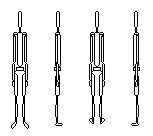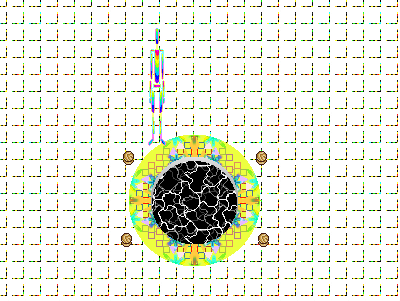Fishing means waiting by the pool with the line in. The longer you wait before pulling the line out, the more likely a creature will appear. Aside from walking, it's the only interaction in the game. The creatures are drawings of things you maybe could find underwater in a dream.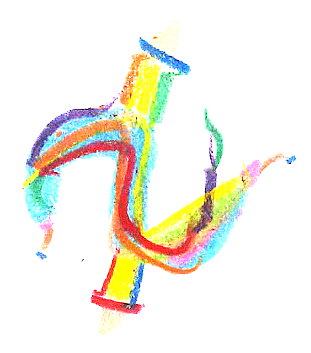The background music is a mix of clips from licensed to share songs on the Free Music Archive. Particularly, Seed64 is an album I used a lot of songs from. The full list of music credits is in the game's README file.

I'm still planning to use the original design in a future version. There would be a reaction-based mini game for catching fish, and the goal would be to catch as many fish as possible within the time limit. I also want to add details and obstacles to the background, which is now a little boring, being a plain, tiled, white floor.

If you want to look at all the drawings or hear the music in the context of the program, there are Windows and source versions available. The source should work on any system with Python and Pygame. If it doesn't, bug reports are much appreciated. Comments are also welcome :)

Dark Stew: Windows, Pygame Source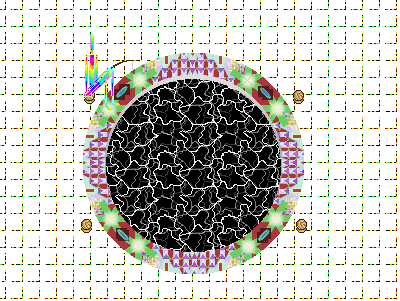I wrote in my last post that I would be working on an old prototype about searching a cloud for organisms for Fishing Jam. I decided to wait a while before developing that game, tentatively titled Xenographic Barrier. Its main interactive element is a first-person scope/flashlight, so I'd like to make a Wii version of it.

I'm about to start working on a complete version of Ball & Cup. If I make anything interesting for it, I'll post something. There are a lot of other things I want to write about, like game analyses, my new GP2X and arcades in Korea, and there's still music to release. Lots of fun stuff coming!